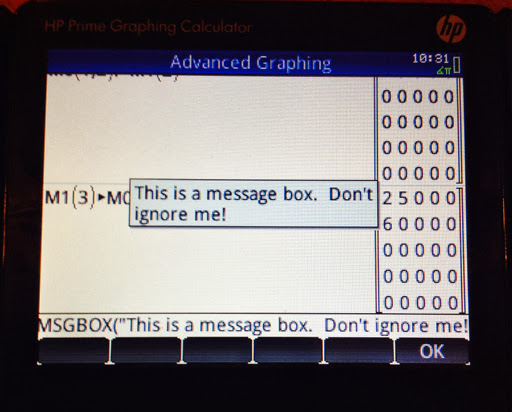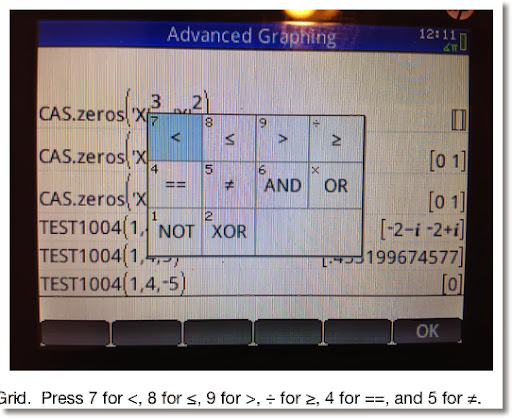## Saturday, October 26, 2013

### HP Prime Programming Tutorial #2: MSGBOX, IF-THEN-ELSE, PRINT, FORWelcome to another programming tutorial for the HP Prime. In this session, we will cover MSGBOX, IF-THEN-ELSE, PRINT, and the FOR loop.

MSGBOX

MSGBOX: MSGOX takes a string a makes a pop-up message box. Program execution stops until you press a key to acknowledge the message.
Access: Cmds, 6. I/O, 8. MSGBOX

The program COMLOCK: Imagine that you are in charge of setting the combinations for the good, old-school combination locks. This program gives three digit combinations through the use of MSGBOX.

EXPORT COMLOCK()
BEGIN
LOCAL L0;
L0:=RANDINT(3,0,39);**
MSGBOX("SECRET: "+L0(1)+","+L0(2)+","+L0(3));
END;

** Thanks to Thomas Lake for pointing out my typo.  Apologies for any inconvenience - Eddie (3/21/2014)

Other commands that are featured:

RANDINT(n, a, b) generates a list of n integers between a and b. You can leave n out if you desire a single random integer. Picks may be repeated.

The HP Prime's default list variables are designated L0 through L9.

Here is a sample output for COMLOCK:IF-THEN-ELSE

IF-THEN-ELSE: Program structure:
IF condition THEN
do if the condition is true;
ELSE
do if the condition is false;
END;

Access: Tmplt, 2. Branch, 2. IF THEN ELSE

Tip: You can leave out the ELSE part if you only want to test to see if a condition is true. Access the simple IF-THEN structure by pressing Tmplt, 2. Branch, 1. IF THEN.

Access <, ≤, ==, etc. by pressing Shift, 6. Note that the double equals is needed to check equality.PRINT

PRINT: The PRINT command prints a sting, result, or a combination of both onto the Prime's Terminal screen. If PRINT is used, the program will end on the terminal (text output) screen. Press a button to exit.

You can access the terminal screen at any time by pressing the ON button, holding it, and then pressing the Divide ( ÷ ) button.

Access: Cmds, 6. I/O, 9. PRINT

Tip: To clear the terminal screen, type PRINT(). This is a good way to clear the terminal screen and I usually use this at the beginning of any program if PRINT is going to be used later on.

The program QROOTS (yet one more quadratic solver, sorry for not being original guys and gals), demonstrates the use of IF-THEN-ELSE and PRINT.

Here I set the setting variable HComplex to 1, which allows for complex number results.

EXPORT QROOTS(A,B,C)
BEGIN
LOCAL D;
PRINT();
HComplex:=1;
D:=B^2-4*A*C;
IF D≥0 THEN
PRINT("Roots are real.");
ELSE
PRINT("Roots are complex.");
END;
PRINT((-B+√D)/(2*A));
PRINT((-B-√D)/(2*A));
END;

Examples:

QROOTS(1,5,8) returns:

Roots are complex.
-2.5+1.32287565553*i
-2.5-1.32287565553*i

QROOTS(2,-4,-8) returns:

Roots are real.
3.2360679775
-1.2360679775

FOR

This section will explore the basic FOR structure:

FOR variable FROM start TO end DO
commands;
END;

All the commands in the loop will be executed a set number of times. Each time a loop finishes, the variable increases by one. The loop terminates when variable=end.

Access: Tmplt, 3. LOOP, 1. FOR

The program SUMDIV takes any integer and adds up the sum of its divisors. For example, the divisors of 12 are 1, 12, 2, 3, 4, and 6. The sum is 28.

Featured Commands in SUMDIV:

idivis: idivis(integer) returns a sequence of all of the divisors if integer. Access: Toolbox, CAS, 5. Integer, 1. Divisors

Any CAS command used in programming will be preceded by "CAS." Not all CAS commands can be used in HP Prime programming at this time.

DIM: returns the dimensions of a sequence, string, or matrix. DIM must be used instead of SIZE to prevent a Bad Argument error.

For sequences or vectors, DIM returns the length in a list {length}.
For strings, DIM returns length as a number.
For matrices, DIM returns the list {number of rows, number of columns}.

Access: Cmds, 1. Strings, 9. DIM

The program:

EXPORT SUMDIV(N)
BEGIN
LOCAL S:=0,K,mdiv,ldiv;
mdiv:=CAS.idivis(N);
ldiv:=DIM(mdiv);
FOR K FROM 1 TO ldiv(1) DO
S:=S+mdiv(K);
END;
RETURN S;
END;
** Thanks to Thomas Lake for pointing out that the variable "mat", which I had in this program was unnecessary.  - Eddie 3/21/2013

Examples:
SUMDIV(12) returns 28.
SUMDIV(24) returns 60.
SUMDIV(85) returns 108.

This concludes this part of the HP Prime Programming Tutorial.

HAPPY HALLOWEEN!

Eddie

This blog is property of Edward Shore. 2013

### RCL 40 Book On Sale

RCL 40 Book On Sale The fantastic book, RCL 40: Recollection, Reinvention, & HP Calculators , edited by W.A.C. Mier-Jedrezejowic, Ph. D,...